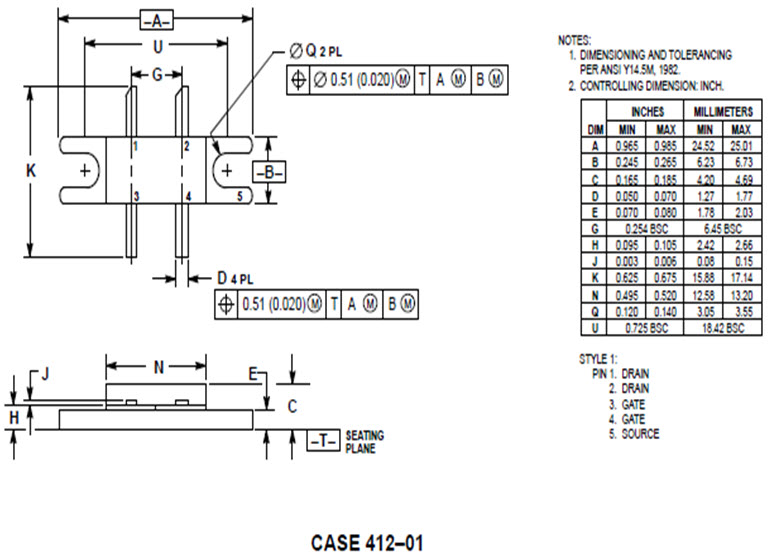# MRF166W - Silicon MOS N-Channel RF Power Transistor 40 W, up to 500 MHz, Enhancement ModeDesigned primarily for wideband large–signal output and driver from 30–500 MHz.

Features:

• Performance at 400 MHz, 28 Vdc
• Power Gain: 11 dB Min
• Output Power: 40 W
• Efficiency: 45 % Min
Send request
Send request

## Absolute Maximum Ratings

Drain-Source Voltage VDSS 65 VDC
Gate-Source Voltage VGS ±40 VDC
Storage Temperature Range TSTG -65 tu +150 ºC
Thermal Resistance, Junction to Case RqJC 1.0 ºC/W
Total Power Dissipation @TC=25 oC PD 175 W

## Parameters

ParameterSymbolMin.Typ.Max.Unit
Drain-Source Breakdown Voltage (ID=5.0 mA, VGS=0 V) V(BR)DSS 65 VDC
Gate-Source Leakage Current (VGS=20 V, VDS=0 V) (1) IGSS 1.0 mADC
Zero Gate Voltage Drain Leakage Current (VDS = 28 V, VGS=0 V) (1) IDSS 1.0 mADC
Gate Threshold Voltage (VDS = 10 V, ID = 25 mA) (1) VGS(TH) 1 6 VDC
Forward Transconductance (VDS = 10 V, ID = 1.5 A) (1) GFS 0.6 0.8 mhos
Input Capacitance (VDS = 28 V, VGS=0 V, f = 1 MHz) (1) CISS 30 pF
Output Capacitance (VDS = 28 V, VGS=0 V, f = 1 MHz) (1) COSS 35 pF
Reverse Transfer Capacitance (VDS = 28 V, VGS=0 V, f = 1 MHz) (1) CRSS 4.5 pF
Power Gain (VDS = 28 V, POUT = 40W, lDQ. = 100 mA,  f = 400 MHz) Gp 11 13 dB
Drain Efficiency (VDS = 28 V, POUT = 40 W, lDQ. = 100 mA,  f = 400 MHz) hD 45 50 %